SELF 36 S.B. Karavashkin and O.N. Karavashkina6. On the invariance of Minkowski 4-D interval When considering in previous items the basic aspects of SR, we directly or indirectly encountered the concept of invariant with which in relativistic conception the Minkowski 4-D interval is associated. Hopefully, it would be not out of Readers’ interest to recall that the 4-D interval per se appeared in SR not from the very beginning. Starting from the first Einstein’s work “On electrodynamics of moving bodies”  and up to his work of 1907 “On the principle of relativity and its corollaries” , all Einstein’s studies were based on variations of Lorentz transformations and their corollaries. Only in his work of 1907 he writes so: “As the speed of light propagation in a void in relation to both coordinate systems is c, the equations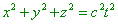(82)
 and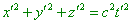(83)
 have to be equivalent” [19, p. 71- 72]. But (82) and (83) as such yet do not mean that he introduces the 4-D interval, they only establish the equivalence of (82) and (83) in relation to IRFs and only for the case when the light propagated in the void space. The concept ‘invariance’ appears in Einstein’s works, beginning with 1911 (i.e. five years after A. Poincare formulated the conditions of invariance of 4-D interval in his famous work “On electron dynamics”), when Einstein formulates, referring to Minkowski, the definition of invariance: “The equations of transformation in the theory of relativity are such that they have the following invariance: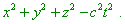(84)
 If instead the time t we introduce as the time coordinate the imaginary variable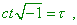(85)
 this invariant takes the form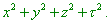(86)
 With it the spatial and temporal coordinates take an equivalent part. The further application of this formal equivalence of spatial and temporal coordinates has led to an extremely clear explanation of the theory of relativity, which makes essentially easier its applications. The physical events are drawn in four-dimensional world and space-time relationships between them are represented in this four-dimensional world by geometrical theorems” [9, p. 186]. Finally, in “The project of generalised theory of relativity and gravity theory” , Einstein , also referring, but now to Planck, writes the principle of least action for SR, basing on 4-D interval: “In accordance with trivial theory of relativity, a free point moves as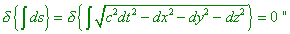(87)
 [20, p. 228]. Thereupon the conceptions of invariant and 4-D complex interval have been unified: “If we take a formal point of view…, we can speak of 4-D space with coordinates xi (i = 1, ..., 4)  whose geometry has to be corresponding to the group of transformations (53); we would mark that the form (54) is invariant with respect to these transformations” [14, p. 93]. At the same time, the concepts of invariant and 4-D interval are basically different. “The 4-D interval is the value characterising the interrelation between the spatial distances and time intervals that separate two events” [21, p. 187]. In its turn, “The metric invariant is such rational function I of the coefficients of equation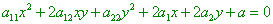(88)
 and of components gik  of metric tensor of the given general Cartesian coordinate system, which has the same value in any two such systems: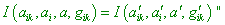(89)
 [22, p. 649]. Or in more general form after Klein: “The numerical characteristics and properties of figures unchanged in some transformation f of the space M are called the invariants of transformation f ” [23, p. 44]. Unification of conceptions of invariant and interval leads only to paradoxes, when, e.g., the interval vanishes in body’s motion with the utmost relativistic speed c. With it, the excuses like “If, moreover, the point moved with the speed of light c, the form (54) will be identically zero and, consequently, the 4-D trajectory of such point will be an isotropic direct line” [14, p. 94- 95] – in no way is able to lift the paradox. With inertial motion of a body in IRF with the speed less than that of light, the trajectory also will be an isotropic direct line. On the contrary, after geometrical axioms, the point cannot have a length; this means, no isotropic direct line is possible - or (54) does not define the trajectory of a body and is only the invariant of relativistic conception. Well, then relativists may not write the principle of least action as (87), with all consequences for both SR and GR. As this is the matter of principle, it needs to be specially considered.

Contents: / 29 / 30 / 31 / 32 / 33 / 34 / 35 / 36 / 37 / 38 / 39 /